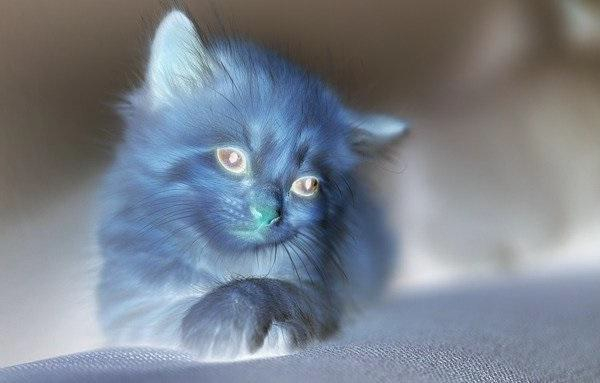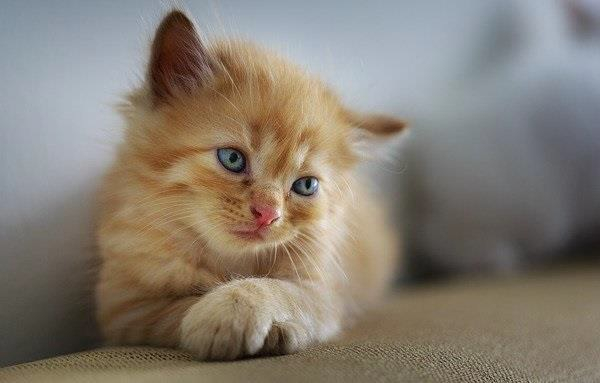# How to convert a negative image to positive image using Java OpenCV library?

To convert a negative image to positive −

• Get the height and width of the image.

• Using nested for loops traverse through each pixel in the image.

• Get the pixel value using the getRGB() method.

• To retrieve each value from a pixel you need to right shift to the beginning position of each color i.e. 24 for alpha 16 for red etc. and perform bitwise and operation with 0Xff. This masks the variable leaving the last 8 bits and ignoring all the rest of the bits.

• Calculate the new red, green and blue values by subtracting them from 255.

• Reconstruct a pixel by shifting the ARGB to left in their respective positions and join them using bitwise OR.

• Set the new pixel value(s) using the setRGB() method.

## Example

import java.io.File;
import java.io.IOException;
import java.awt.image.BufferedImage;
import javax.imageio.ImageIO;
public class Negative2Color {
public static void main(String args[])throws IOException {
File file= new File("D:\Images\cat_neg.jpg");
for (int y = 0; y < img.getHeight(); y++) {
for (int x = 0; x < img.getWidth(); x++) {
//Retrieving contents of a pixel
int p = img.getRGB(x,y);
//Getting the A R G B values from the pixel value
int a = (p>>24)&0xff;
int r = (p>>16)&0xff;
int g = (p>>8)&0xff;
int b = p&0xff;
//Subtract RGB from 255
r = 255 - r;
g = 255 - g;
b = 255 - b;
//Set new RGB value
p = (a<<24) | (r<<16) | (g<<8) | b;
img.setRGB(x, y, p);
}
}
//Saving the modified image
file = new File("D:\Images\negative_positive.jpg");
ImageIO.write(img, "jpg", file);
System.out.println("Done...");
}
}

## Input## Output